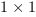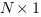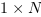Scilab Home page | Wiki | Bug tracker | Forge | Mailing list archives | ATOMS | File exchange
Change language to: Français - Português - 日本語

See the recommended documentation of this function

Scilab help >> Xcos > palettes > Sources palette > STEP_FUNCTION

# STEP_FUNCTION

Step Function

### Block Screenshot### Description

In its simpler use this block output a step signal between two definable levels Initial Value and Final Value starting at a specified time Step Time.

The step function is defined by the following equation:where `t0` is the Start Time, `s0` the Initial Value and `sf` the Final Value.

You can also specify vector or matrice values, for the parameters Initial Value and Final Value. The parameters are expanded to column vectors. and in this case, you obtain multiples output that start at the same Step Time.

The table below shows different combinations

 Initial value size Final value size Output size Condition### Data types

• Output: scalar or column vector of double data type. The size is determined by the parameters Initial Value and Final Value

### Dialog box• Step Time

The time, in seconds, when the output jumps from the Initial Value parameter to the Final value parameter.

Properties : Type 'vec' of size 1.

• Initial Value

The block output `i` is equal to the `ith` Initial Value until the simulation time reaches the Step Time parameter.

Properties : Type 'vec' of size -1.

• Final Value

The block output `i` jump to `ith` Final Value when the simulation time reaches the Step Time parameter.

Properties : Type 'vec' of size -1.

### Default properties

• always active: no

• direct-feedthrough: no

• zero-crossing: no

• mode: no

• regular outputs:

- port 1 : size [-1,1] / type 1

• number/sizes of activation inputs: 0

• number/sizes of activation outputs: 0

• continuous-time state: no

• discrete-time state: no

• object discrete-time state: no

• name of computational function: csuper

### Example 1

This diagram shows the use of the STEP_FUNCTION block to obtain the response to a step signal of a first order's system defined by a transfer function. Open this example in Xcos.### Example 2

This diagram shows the use of STEP_FUNCTION to obtain four step signals Open this example in Xcos.### Authors

Ramine Nikoukhah - INRIA